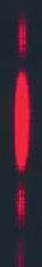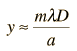# Fraunhofer Single SlitThe diffraction pattern at the right is taken with a helium-neon laser and a narrow single slit.

 More conceptual details about single slit diffraction

The active formula below can be used to model the different parameters which affect diffraction through a single slit. Enter the available measurements or model parameters and then click on the parameter you wish to calculate.

Displacement y = (Order m x Wavelength x Distance D)/(slit width a)

For a slit of width a = micrometers = x10^ m

 and light wavelength λ = nm at order m =,

on a screen at distance D = cm

the displacement from the centerline for minimum intensity will be= cm.

 This corresponds to a diffraction angle of θ = ° .

This calculation is designed to allow you to enter data and then click on the quantity you wish to calculate in the active formula above. The data will not be forced to be consistent until you click on a quantity to calculate. Default values will be entered for unspecified parameters, but all values may be changed.

Note: The small angle approximation was not used in the calculations above, but it is usually sufficiently accurate for laboratory calculations.

 Fraunhofer geometry Intensity for single slit Examine approximation Double slit interference Comparison to double slit
Index

Diffraction concepts

Fraunhofer diffraction

 HyperPhysics***** Light and Vision R Nave
Go Back